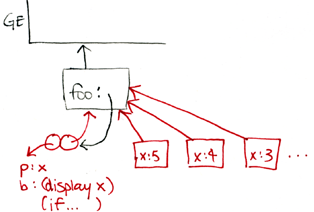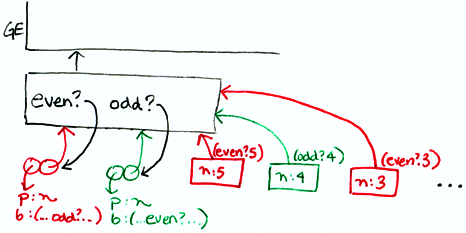```recitation 21: more evaluator practice

--------------------------------------------------------------------
suppose we change the call in m-apply from:
(extend-environment (procedure-parameters procedure)
arguments
(procedure-environment procedure))
to:
(extend-environment (procedure-parameters procedure)
arguments
the-global-environment)

* does this change how we draw environment diagrams?  how?

yes, now all frames will hang directly from the global environment.
the environment pointer of the procedure is ignored.

* write the simplest expression (or set of expressions) you can, which
evaluate to a different value with the original m-apply versus the
modified m-apply.

(define x 5)
((lambda (x) x) 2)

original m-apply --> 2
modified m-apply --> 5

NOTE:  the value of the sugared version of this expression:
(let ((x 2)) x)
will depend on the implementation of let in the evaluator.  (why?)

--------------------------------------------------------------------
(incr! <var> <exp>)

(define x 10)
(incr! x 3)
x --> 13

* change the evaluator to add incr!

* now add decr! does it have to be a special form?

yes decr! must be a special form.  we can't let the application
clause handle this expression, because we don't want to evaluate <var>.

* make a (general) list of the things that need to be changed/added
when you extend the evaluator to add a new special form.

- write expression detector, accessor & constructor functions
- write necessary helper functions
- modify m-apply or other parts of the evaluator, as necessary

--------------------------------------------------------------------
practice using the "named-let" special form:

(let <var> <bindings> <body>)

The <bindings> and <body> are just as in ordinary let, except that
<var> is bound within <body> to a procedure whose body is <body> and
whose parameters are the variables in the <bindings>. Thus, one can
repeatedly execute the <body> by invoking the procedure named <var>.

(let foo ((x 5))
(display x)
(if (< x 1)
'done
(foo (- x 1))))

* draw an environment diagram for this example.  what variables does
``````  NOTE: this is one possible solution.  there are other variations
that also correctly implement the specified semantics.

* how would you use named-let to iteratively reverse a list?
(hint: use 2 variables in the <bindings>)

(let reverse ((rest '(1 2 3 4))
(if (null? rest)
(reverse (cdr rest) (cons (car rest) answer))))

* you're asked to add named-let to the evaluator for project 5...

--------------------------------------------------------------------
practice using the "letrec" special form:

(letrec ((<var1> <exp1>) (<var2> <exp2>) ... ) <body>)

the <var>s are bound in a new frame to (initially) undefined values.
the <exp>s are evaluated in the resulting environment (in unspecified
order), and bound to the corresponding <var>.  the <body> is evaluated
in the resulting environment, and the value of the last expression in
<body> is returned.  each binding of a <var> has the entire `letrec'
expression as its region, making it possible to define mutually
recursive procedures.

* what does it mean to say that m-eval and m-apply are
"mutually-recursive procedures"?

even? calls odd?, and odd? calls even?  the environment
for each procedure must contain the definition for the other.

One restriction on "letrec" is very important: it must be possible to
evaluate each <exp> without assigning or referring to the value of any
<var>. If this restriction is violated, then it is an error. The
restriction is necessary because Scheme passes arguments by value
rather than by name. In the most common uses of `letrec', all the
<exp>s are lambda expressions and the restriction is satisfied
automatically.

(letrec ((even?
(lambda (n)
(if (zero? n)
#t
(odd? (- n 1)))))
(odd?
(lambda (n)
(if (zero? n)
#f
(even? (- n 1))))))
(even? 5))

* draw an environment diagram for this example.  what variables do
``````* use letrec to create a toy expression with 3 mutually-recursive
procedures.  show that your expression does not result in an
infinite loop.

* you're asked to add letrec to the evaluator for project 5...

--------------------------------------------------------------------

* can you rewrite (desugar) a "named-let" using "let"?

* can you rewrite (desugar) a "named-let" using "letrec"?

--------------------------------------------------------------------
(dolist (<var> <lst>) <body>)

<var> is bound to each element in <lst> sequentially and <body> is
executed once for each binding.  the expression returns the value of
the last expression in body for the binding of <var> to the last
element of <lst>.

(dolist (x '(1 2 3 4)) (display (* x x)))

* is dolist a special form?  why or why not?

* change the evaluator to add dolist

--------------------------------------------------------------------
```